# 20.1 Hydrocarbons  (Page 10/22)

 Page 10 / 22

Explain why unbranched alkenes can form geometric isomers while unbranched alkanes cannot. Does this explanation involve the macroscopic domain or the microscopic domain?

Unbranched alkanes have free rotation about the C–C bonds, yielding all orientations of the substituents about these bonds equivalent, interchangeable by rotation. In the unbranched alkenes, the inability to rotate about the $\text{C}=\text{C}$ bond results in fixed (unchanging) substituent orientations, thus permitting different isomers. Since these concepts pertain to phenomena at the molecular level, this explanation involves the microscopic domain.

Explain why these two molecules are not isomers: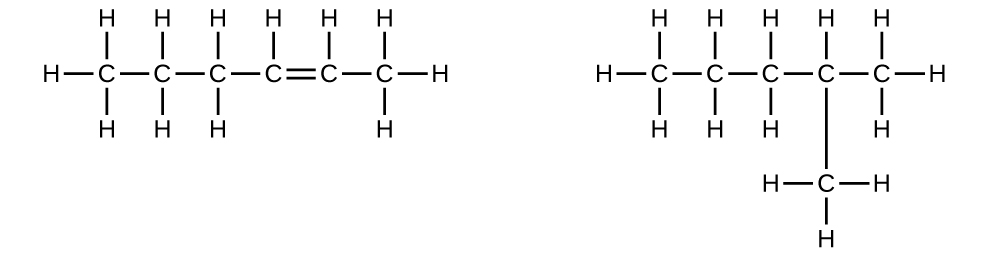Explain why these two molecules are not isomers: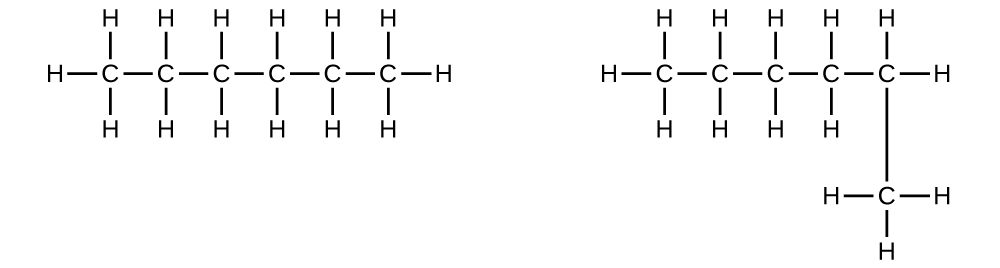They are the same compound because each is a saturated hydrocarbon containing an unbranched chain of six carbon atoms.

How does the carbon-atom hybridization change when polyethylene is prepared from ethylene?

Write the Lewis structure and molecular formula for each of the following hydrocarbons:

(a) hexane

(b) 3-methylpentane

(c) cis -3-hexene

(d) 4-methyl-1-pentene

(e) 3-hexyne

(f) 4-methyl-2-pentyne

(a) C 6 H 14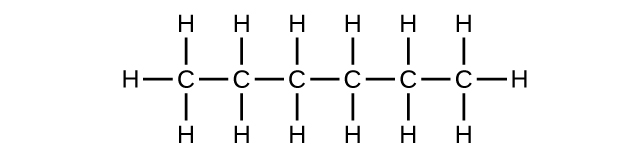;
(b) C 6 H 14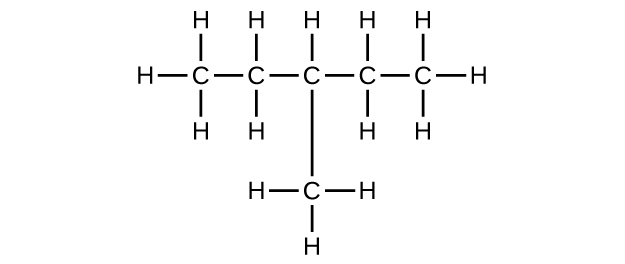;
(c) C 6 H 12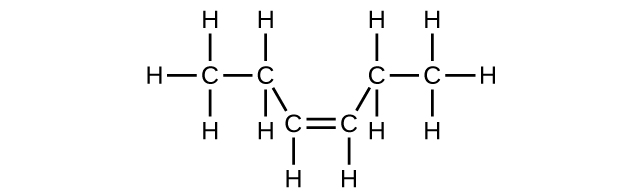;
(d) C 6 H 12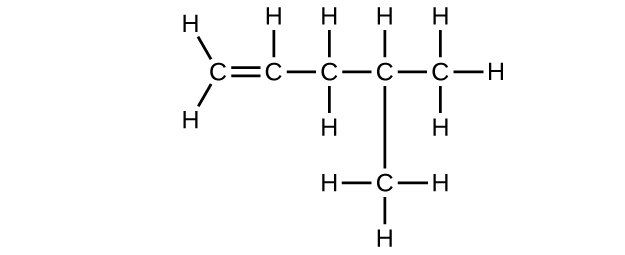;
(e) C 6 H 10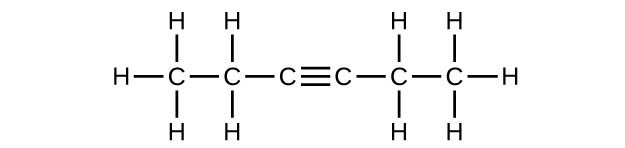;
(f) C 6 H 10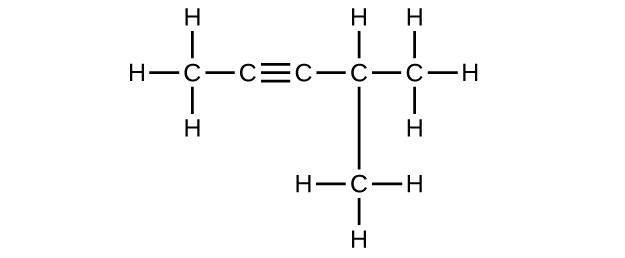Write the chemical formula, condensed formula, and Lewis structure for each of the following hydrocarbons:

(a) heptane

(b) 3-methylhexane

(c) trans -3-heptene

(d) 4-methyl-1-hexene

(e) 2-heptyne

(f) 3,4-dimethyl-1-pentyne

Give the complete IUPAC name for each of the following compounds:

(a) CH 3 CH 2 CBr 2 CH 3

(b) (CH 3 ) 3 CCl

(c)(d) ${\text{CH}}_{3}{\text{CH}}_{2}\text{C}\equiv {\text{CH CH}}_{3}{\text{CH}}_{2}\text{C}\equiv \text{CH}$

(e)(f)(g) ${\left({\text{CH}}_{3}\right)}_{2}{\text{CHCH}}_{2}\text{CH}={\text{CH}}_{2}$

(a) 2,2-dibromobutane; (b) 2-chloro-2-methylpropane; (c) 2-methylbutane; (d) 1-butyne; (e) 4-fluoro-4-methyl-1-octyne; (f) trans -1-chloropropene; (g) 5-methyl-1-pentene

Give the complete IUPAC name for each of the following compounds:

(a) (CH 3 ) 2 CHF

(b) CH 3 CHClCHClCH 3

(c)(d) ${\text{CH}}_{3}{\text{CH}}_{2}\text{CH}={\text{CHCH}}_{3}$

(e)(f) ${\left({\text{CH}}_{3}\right)}_{3}{\text{CCH}}_{2}\text{C}\equiv \text{CH}$

Butane is used as a fuel in disposable lighters. Write the Lewis structure for each isomer of butane.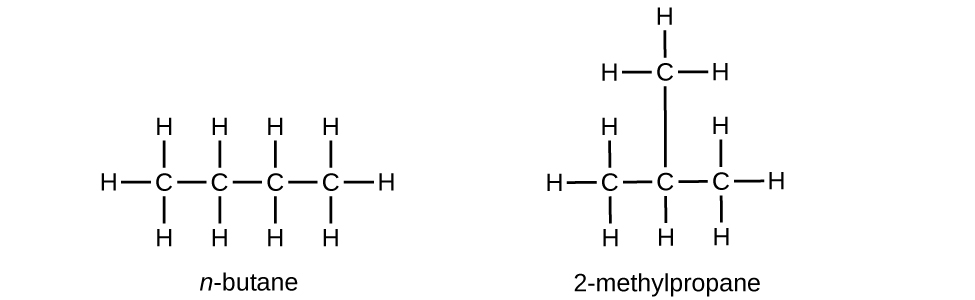Write Lewis structures and name the five structural isomers of hexane.

Write Lewis structures for the cis–trans isomers of ${\text{CH}}_{3}\text{CH}=\text{CHCl.}$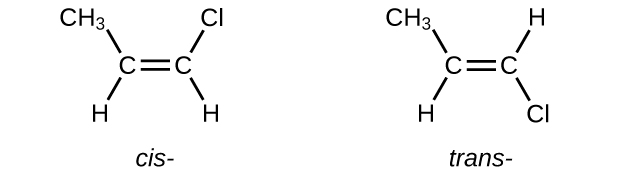Write structures for the three isomers of the aromatic hydrocarbon xylene, C 6 H 4 (CH 3 ) 2 .

Isooctane is the common name of the isomer of C 8 H 18 used as the standard of 100 for the gasoline octane rating:(a) What is the IUPAC name for the compound?

(b) Name the other isomers that contain a five-carbon chain with three methyl substituents.

(a) 2,2,4-trimethylpentane; (b) 2,2,3-trimethylpentane, 2,3,4-trimethylpentane, and 2,3,3-trimethylpentane: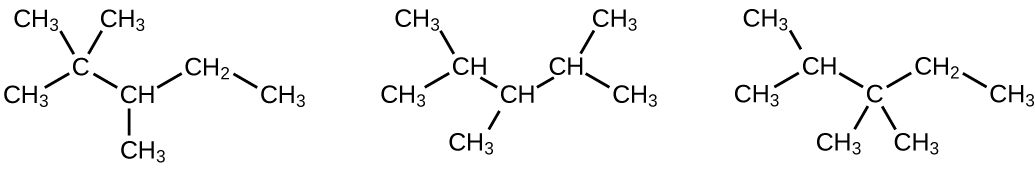Write Lewis structures and IUPAC names for the alkyne isomers of C 4 H 6 .

Write Lewis structures and IUPAC names for all isomers of C 4 H 9 Cl.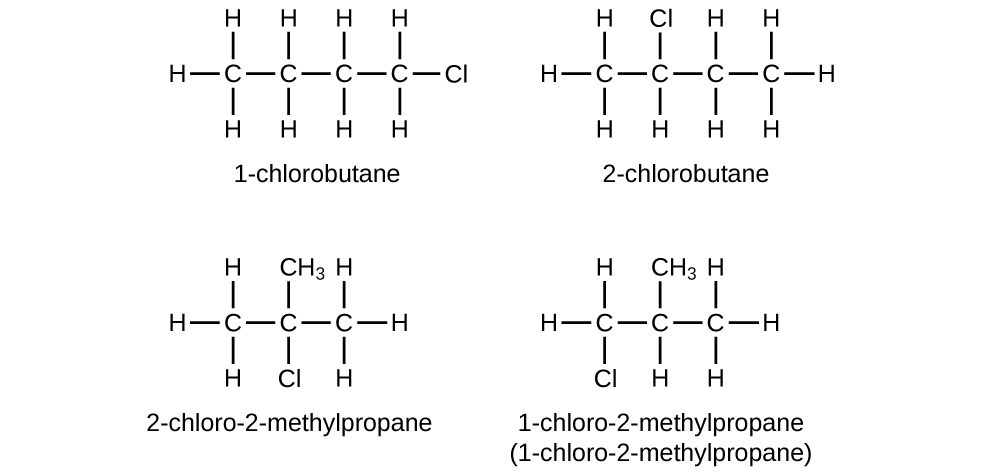Name and write the structures of all isomers of the propyl and butyl alkyl groups.

Write the structures for all the isomers of the –C 5 H 11 alkyl group.

In the following, the carbon backbone and the appropriate number of hydrogen atoms are shown in condensed form: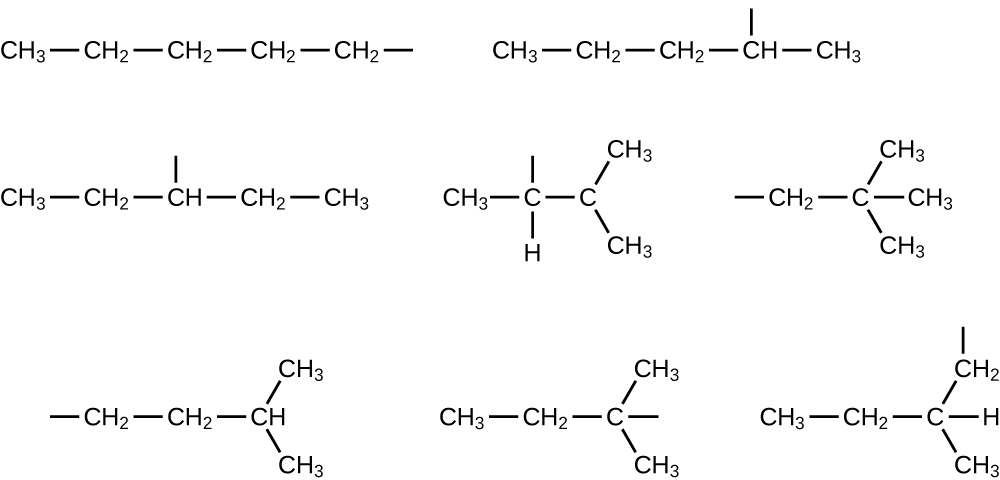Write Lewis structures and describe the molecular geometry at each carbon atom in the following compounds:

(a) cis -3-hexene

(b) cis -1-chloro-2-bromoethene

(c) 2-pentyne

(d) trans - 6 -ethyl-7-methyl-2-octene

Benzene is one of the compounds used as an octane enhancer in unleaded gasoline. It is manufactured by the catalytic conversion of acetylene to benzene:
$3{\text{C}}_{2}{\text{H}}_{2}\phantom{\rule{0.2em}{0ex}}⟶\phantom{\rule{0.2em}{0ex}}{\text{C}}_{6}{\text{H}}_{6}$

Draw Lewis structures for these compounds, with resonance structures as appropriate, and determine the hybridization of the carbon atoms in each.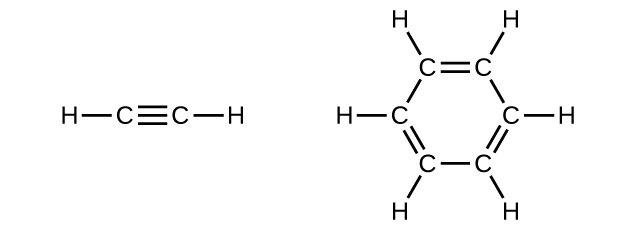In acetylene, the bonding uses sp hybrids on carbon atoms and s orbitals on hydrogen atoms. In benzene, the carbon atoms are sp 2 hybridized.

Teflon is prepared by the polymerization of tetrafluoroethylene. Write the equation that describes the polymerization using Lewis symbols.

Write two complete, balanced equations for each of the following reactions, one using condensed formulas and one using Lewis structures.

(a) 1 mol of 1-butyne reacts with 2 mol of iodine.

(b) Pentane is burned in air.

(a) $\text{CH}\equiv {\text{CCH}}_{2}{\text{CH}}_{3}+2{\text{I}}_{2}\phantom{\rule{0.2em}{0ex}}⟶\phantom{\rule{0.2em}{0ex}}{\text{CHI}}_{2}{\text{CI}}_{2}{\text{CH}}_{2}{\text{CH}}_{3}$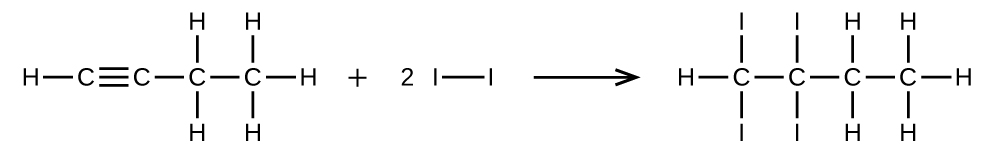;
(b) ${\text{CH}}_{3}{\text{CH}}_{2}{\text{CH}}_{2}{\text{CH}}_{2}{\text{CH}}_{3}+8{\text{O}}_{2}\phantom{\rule{0.2em}{0ex}}⟶\phantom{\rule{0.2em}{0ex}}5{\text{CO}}_{2}+{\text{6H}}_{2}\text{O}$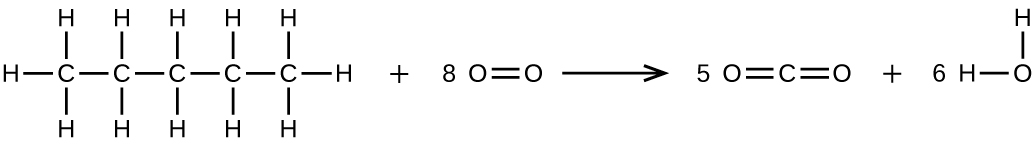Write two complete, balanced equations for each of the following reactions, one using condensed formulas and one using Lewis structures.

(a) 2-butene reacts with chlorine.

(b) benzene burns in air.

What mass of 2-bromopropane could be prepared from 25.5 g of propene? Assume a 100% yield of product.

65.2 g

Acetylene is a very weak acid; however, it will react with moist silver(I) oxide and form water and a compound composed of silver and carbon. Addition of a solution of HCl to a 0.2352-g sample of the compound of silver and carbon produced acetylene and 0.2822 g of AgCl.

(a) What is the empirical formula of the compound of silver and carbon?

(b) The production of acetylene on addition of HCl to the compound of silver and carbon suggests that the carbon is present as the acetylide ion, ${\text{C}}_{2}{}^{2-}$ . Write the formula of the compound showing the acetylide ion.

Ethylene can be produced by the pyrolysis of ethane:
${\text{C}}_{2}{\text{H}}_{6}\phantom{\rule{0.2em}{0ex}}⟶\phantom{\rule{0.2em}{0ex}}{\text{C}}_{2}{\text{H}}_{4}+{\text{H}}_{2}$

How many kilograms of ethylene is produced by the pyrolysis of 1.000 $×$ 10 3 kg of ethane, assuming a 100.0% yield?

9.328 $×$ 10 2 kg

following processes: Solid phosphorus pentachloride decomposes to liquid phosphorus trichloride and chlorine gas b. Deep blue solid copper(II) sulfate pentahydrate is heated to drive off water vapor to form white solid copper(II) sulfate
How to know periodic table oftend
how to get atomic number of an element
how do you solve the examples in a much more explanatory way
Ogunleye
The reaction of aceto nitrile with propane in the presence of the acid
Explain this paragraph in short
What is solid state?
What is chemical reaction
Manish
transforming reactants to product(s)
Andre
process
Andre
Example of Lewis acid
Example of Lewis acid
Chidera
Chlorine
Anything with an empty orbital... the hydrogen ion is the most common example. BH3 is the typical example, but any metal in a coordination complex can be considered a Lewis acid.
Eszter
okay thanks
Jovial
aluminium and sulphur react to give aluminium sulfide.How many grams of Al are required to produce 100g of aluminium sulphide
aluminium and sulphur react to give aluminium sulphide how many grams of Al are required to produce 100g of aluminium sulphide?
Soni
aluminium and sulphur react to give aluminium sulphide how many grams of Al are required to produce 100g of aluminium sulphide?
Soni
2Al+3S=Al2S3
galina
m(Al)=100×27×2/150=36g
galina
150 comes from?
Soni
thank you very much
Soni
molar mass of Al2S3
galina
150.158
thiru
Why can't atom be created or destroyed
matter simply converts to pure energy
that's nice
Meshach
explain how to distinguish ethanol from a sample of ethanoic acid by chemical test
explain how ethanol can be distinguished from ethanoic acid by chemical test
Alice
Using a suitable experiment, describe how diffusion occurs in gases.
when the excited energy which are in gaseous state collides with another to liberate from one place to another
Meshach
what is electrolytes?
substance which splits into ions during melting or dissolving
galina
on passing electric current though electrode
Kv
State that use law of partial pressure in a gas jar containing a gas and water what is the total pressure composed of 272cm^3 of carbon (iv) oxide were collected over water at15°c and 782mmHg pressure. calculate the volume of the dry gas at stp(SVP of water at 15°c is 12mmHg)
was Dalton's second postulate"atoms of the same kind have have similar/same mass and size" Or " the one mentioned in B here?ByByBy Robert MorrisBy Brooke DelaneyBy OpenStaxBy OpenStaxBy OpenStaxBy Tess ArmstrongBy OpenStaxBy Miranda ReisingBy OpenStaxBy OpenStax